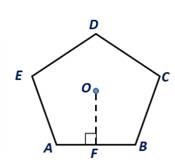Chapter 8.CT, Problem 9CT### Elementary Geometry for College St...

6th Edition
Daniel C. Alexander + 1 other
ISBN: 9781285195698

#### Solutions

Chapter
Section### Elementary Geometry for College St...

6th Edition
Daniel C. Alexander + 1 other
ISBN: 9781285195698
Textbook Problem
1 views

# A regular pentagon has an apothem of length 4.0 in. and each side is of length s=5.8 in. For the regular pentagon, find its:a) Perimeter                                   _ b) Area                                   _To determine

a.

To find:

The perimeter of the regular pentagon.

Explanation

Given:

A regular pentagon has an apothem of length 4.0 in. and each side is of length 5.8 in. For the regular pentagon.

Definition:

For a regular polygon of n sides, each side of length s, its perimeter is P=ns.

Calculation:

Given that the length of the side of the regular pentagon is 5

To determine

b.

To find:

The area of the regular pentagon.

### Still sussing out bartleby?

Check out a sample textbook solution.

See a sample solution

#### The Solution to Your Study Problems

Bartleby provides explanations to thousands of textbook problems written by our experts, many with advanced degrees!

Get Started

#### Find an equation of the line that passes through (1, 2) and (4, 5).

Applied Calculus for the Managerial, Life, and Social Sciences: A Brief Approach

#### A sample with a mean of M = 8 has X = 56. How many scores are in the sample?

Essentials of Statistics for The Behavioral Sciences (MindTap Course List)

#### The normal plane to at t = 1 has equation:

Study Guide for Stewart's Multivariable Calculus, 8th

#### f(x) = x3 2x2 + x 1 has a local maximum at: a) 0 b) 1 c) 13 d) f has no local maxima

Study Guide for Stewart's Single Variable Calculus: Early Transcendentals, 8th